# boolean algebra

## Introduction

Mathematical rules are based on the defining limits we place on the particular numerical quantities dealt with. When we say that 1 + 1 = 2 or 3 + 4 = 7, we are implying the use of integerquantities: the same types of numbers we all learned to count in elementary education. What most people assume to be self-evident rules of arithmetic — valid at all times and for all purposes — actually depend on what we define a number to be.

For instance, when calculating quantities in AC circuits, we find that the “real” number quantities which served us so well in DC circuit analysis are inadequate for the task of representing AC quantities. We know that voltages add when connected in series, but we also know that it is possible to connect a 3-volt AC source in series with a 4-volt AC source and end up with 5 volts total voltage (3 + 4 = 5)! Does this mean the inviolable and self-evident rules of arithmetic have been violated? No, it just means that the rules of “real” numbers do not apply to the kinds of quantities encountered in AC circuits, where every variable has both a magnitude and a phase. Consequently, we must use a different kind of numerical quantity, or object, for AC circuits (complex numbers, rather than real numbers), and along with this different system of numbers comes a different set of rules telling us how they relate to one another.

An expression such as “3 + 4 = 5” is nonsense within the scope and definition of real numbers, but it fits nicely within the scope and definition of complex numbers (think of a right triangle with opposite and adjacent sides of 3 and 4, with a hypotenuse of 5). Because complex numbers are two-dimensional, they are able to “add” with one another trigonometrically as single-dimension “real” numbers cannot.

Logic is much like mathematics in this respect: the so-called “Laws” of logic depend on how we define what a proposition is. The Greek philosopher Aristotle founded a system of logic based on only two types of propositions: true and false. His bivalent (two-mode) definition of truth led to the four foundational laws of logic: the Law of Identity (A is A); the Law of Non-contradiction (A is not non-A); the Law of the Excluded Middle (either A or non-A); and the Law of Rational Inference. These so-called Laws function within the scope of logic where a proposition is limited to one of two possible values, but may not apply in cases where propositions can hold values other than “true” or “false.” In fact, much work has been done and continues to be done on “multivalued,” or fuzzy logic, where propositions may be true or false to a limited degree. In such a system of logic, “Laws” such as the Law of the Excluded Middle simply do not apply, because they are founded on the assumption of bivalence. Likewise, many premises which would violate the Law of Non-contradiction in Aristotelian logic have validity in “fuzzy” logic. Again, the defining limits of propositional values determine the Laws describing their functions and relations.

The English mathematician George Boole (1815-1864) sought to give symbolic form to Aristotle’s system of logic. Boole wrote a treatise on the subject in 1854, titled An Investigation of the Laws of Thought, on Which Are Founded the Mathematical Theories of Logic and Probabilities, which codified several rules of relationship between mathematical quantities limited to one of two possible values: true or false, 1 or 0. His mathematical system became known as Boolean algebra.

All arithmetic operations performed with Boolean quantities have but one of two possible outcomes: either 1 or 0. There is no such thing as “2” or “-1” or “1/2” in the Boolean world. It is a world in which all other possibilities are invalid by fiat. As one might guess, this is not the kind of math you want to use when balancing a checkbook or calculating current through a resistor. However, Claude Shannon of MIT fame recognized how Boolean algebra could be applied to on-and-off circuits, where all signals are characterized as either “high” (1) or “low” (0). His 1938 thesis, titled A Symbolic Analysis of Relay and Switching Circuits, put Boole’s theoretical work to use in a ay Boole never could have imagined, giving us a powerful mathematical tool for designing and analyzing digital circuits.

In this chapter, you will find a lot of similarities between Boolean algebra and “normal” algebra, the kind of algebra involving so-called real numbers. Just bear in mind that the system of numbers defining Boolean algebra is severely limited in terms of scope, and that there can only be one of two possible values for any Boolean variable: 1 or 0. Consequently, the “Laws” of Boolean algebra often differ from the “Laws” of real-number algebra, making possible such statements as 1 + 1 = 1, which would normally be considered absurd. Once you comprehend the premise of all quantities in Boolean algebra being limited to the two possibilities of 1 and 0, and the general philosophical principle of Laws depending on quantitative definitions, the “nonsense” of Boolean algebra disappears.

It should be clearly understood that Boolean numbers are not the same as binary numbers. Whereas Boolean numbers represent an entirely different system of mathematics from real numbers, binary is nothing more than an alternative notation for real numbers. The two are often confused because both Boolean math and binary notation use the same two ciphers: 1 and 0. The difference is that Boolean quantities are restricted to a single bit (either 1 or 0), whereas binary numbers may be composed of many bits adding up in place-weighted form to a value of any finite size. The binary number 100112 (“nineteen”) has no more place in the Boolean world than the decimal number 210 (“two”) or the octal number 328 (“twenty-six”).

0 + 0 = 0

0 + 1 = 1

1 + 0 = 1

1 + 1 = 1

## Boolean arithmetic

Let us begin our exploration of Boolean algebra by adding numbers together:The first three sums make perfect sense to anyone familiar with elementary addition. The last sum, though, is quite possibly responsible for more confusion than any other single statement in digital electronics, because it seems to run contrary to the basic principles of mathematics. Well, it does contradict principles of addition for real numbers, but not for Boolean numbers. Remember that in the world of Boolean algebra, there are only two possible values for any quantity and for any arithmetic operation: 1 or 0. There is no such thing as “2” within the scope of Boolean values. Since the sum “1 + 1” certainly isn’t 0, it must be 1 by process of elimination.

It does not matter how many or few terms we add together, either. Consider the following sums: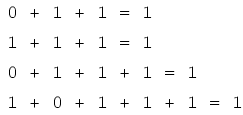Take a close look at the two-term sums in the first set of equations. Does that pattern look familiar to you? It should! It is the same pattern of 1’s and 0’s as seen in the truth table for an OR gate. In other words, Boolean addition corresponds to the logical function of an “OR” gate, as well as to parallel switch contacts: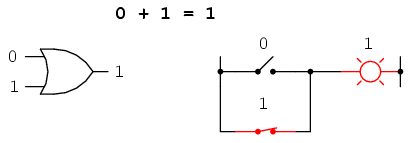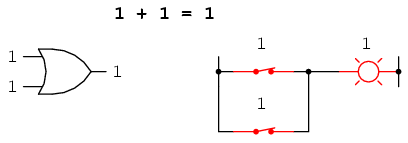There is no such thing as subtraction in the realm of Boolean mathematics. Subtraction implies the existence of negative numbers: 5 – 3 is the same thing as 5 + (-3), and in Boolean algebra negative quantities are forbidden. There is no such thing as division in Boolean mathematics, either, since division is really nothing more than compounded subtraction, in the same way that multiplication is compounded addition.

Multiplication is valid in Boolean algebra, and thankfully it is the same as in real-number algebra: anything multiplied by 0 is 0, and anything multiplied by 1 remains unchanged: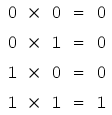This set of equations should also look familiar to you: it is the same pattern found in the truth table for an AND gate. Inother words, Boolean multiplication corresponds to the logical function of an “AND” gate, as well as to series switch contacts: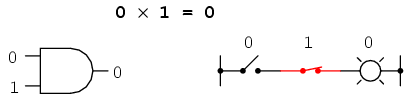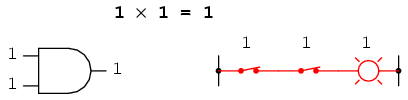Like “normal” algebra, Boolean algebra uses alphabetical letters to denote variables. Unlike “normal” algebra, though, Boolean variables are always CAPITAL letters, never lower-case. Because they are allowed to possess only one of two possible values, either 1 or 0, each and every variable has a complement: the opposite of its value. For example, if variable “A” has a value of 0, then the complement of A has a value of 1. Boolean notation uses a bar above the variable character to denote complementation, like this:In written form, the complement of “A” denoted as “A-not” or “A-bar”. Sometimes a “prime” symbol is used to represent complementation. For example, A’ would be the complement of A, much the same as using a prime symbol to denote differentiation in calculus rather than the fractional notation d/dt. Usually, though, the “bar” symbol finds more widespread use than the “prime” symbol, for reasons that will become more apparent later in this chapter.

Boolean complementation finds equivalency in the form of the NOT gate, or a normally-closed switch or relay contact:The basic definition of Boolean quantities has led to the simple rules of addition and multiplication, and has excluded both subtraction and division as valid arithmetic operations. We have a symbology for denoting Boolean variables, and their complements. In the next section we will proceed to develop Boolean identities.

• REVIEW:
• Boolean addition is equivalent to the OR logic function, as well as parallel switch contacts.
• Boolean multiplication is equivalent to the AND logic function, as well as series switch contacts.
• Boolean complementation is equivalent to the NOT logic function, as well as normally-closed relay contacts.

Related

COMMENT songs